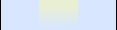WhiteSpace sourcehome  ·  contact

# WhiteSpace

## Calculator

This is the second release of our WhiteSpace Scientific Calculator. It features programmable functions, variables and a range of standard mathematical functions.

Current build: september, 21th, 2001

The upper TextArea displays the memory of the applet. It is empty at start, but will show the variables and functions you define.

The area below shows the answers. You see that all answers are numbered: you can recall them by typing ans([number]).

Under the output area is the input field. You can type here whatever you want to have calculated. If you press enter in this field, the calculator calculates.

You can use the keypad to enter symbols with the mouse. The symbols will appear in the input field. You need to press OK if you want to calculate the input. On other calculators you can use the = button to do that, but that button is used to store variables here.

## Examples

3+2*5
calculates 13. note that the calculator places the ( ) like you want them.
x=2.22**sqrt(2)
This sets the variable x. If it already had a value, it is overwritten. ** is used for exponentiation. The ^ symbol denotes bitwise XOR, just like in java
x==0||x<=10
Comparisions return 1.0 if true, 0.0 if false. You can make complex conditions by using the standard boolean operators: ||, &&, !. Note that the double == is the equality operator, not =.
ans(11)*ans(12)
This multiplies the 11th and 12th answer. Everything you calculate is stored.
fac(x)=if(x<=0,1,x*fac(x-1))
Calculates the faculty function. You define functions just like variables, with the = operator. A definition return 1.0 is succesful.

## Reference

abs(a0)
return the distance to zero
abs(a0)
return the distance to zero
and(a0,a1)
do bitwise and on two numbers
and(a0,a1)
do bitwise and on two numbers
and(a0,a1,a2,a3,a4,a5)
returns true if both arguments are true
and(a0,a1,a2,a3,a4,a5)
returns true if both arguments are true
andb(a0,a1)
bitwise and
andb(a0,a1)
bitwise and
ans(a0)
bi_one()
return bigInteger with value 1
bi_zero()
return bigInteger with value 0
ceil(a0)
round to a higher integer
ceil(a0)
round to a higher integer
codeblock(a0,a1,a2,a3,a4,a5)
do these commands
cos(a0)
cosinus function
cos(a0)
cosinus function
div(a0,a1)
divide two bigInts
div(a0,a1)
divide one integer by another
div(a0,a1)
divide two bigInts
eq(a0,a1)
returns true if the arguments are equal
eq(a0,a1)
returns true if the arguments are equal
euler()
returns the number e=2.71828...
exp(a0,a1)
raise a0 to the power a1
exp(a0,a1)
raise a1 to the power a2
exp(a0,a1)
raise a0 to the power a1
floor(a0)
round to a lower integer
floor(a0)
round to a lower integer
gcd(a0,a1)
get greatest common divisor of two bigints
gcd(a0,a1)
get greatest common divisor of two bigints
goodbye(a0)
destroy local variable
gt(a0,a1)
returns true if the first number is greater than the second
gt(a0,a1)
returns true if the first number is greater than the second
gte(a0,a1)
returns true if the first number is not less
gte(a0,a1)
returns true if the first number is not less
help(a0)
get help on command
if(a0,a1)
if a0 is true do a1
if(a0,a1,a2)
if a0 is true do a1 else do a2
isprime(a0)
returns true if bigint a0 is prime
isprime(a0)
returns true if bigint a0 is prime
length(a0)
return the number of bits of a0
length(a0)
return the number of bits of a0
let(a0,a1)
define function
log(a0)
calculate the natural logarithm
log(a0)
calculate the natural logarithm
lshift(a0,a1)
shift a number to the left
lshift(a0,a1)
shift a number to the left
lt(a0,a1)
returns true if the first number is less than the second
lt(a0,a1)
returns true if the first number is less than the second
lte(a0,a1)
returns true if the first number is not greater
lte(a0,a1)
returns true if the first number is not greater
makemanual(a0)
make manual file
mod(a0,a1)
return the remainder of a0 from division by a1
mod(a0,a1)
calculate the remainder
mod(a0,a1)
return the remainder of a0 from division by a1
modexp(a0,a1,a2)
return (a0**a1)%a2
modexp(a0,a1,a2)
return (a0**a1)%a2
modinv(a0,a1)
get the inverse of a0 in Z_a1
modinv(a0,a1)
get the inverse of a0 in Z_a1
mul(a0,a1)
divide two BigInts
mul(a0,a1)
divide two BigInts
mul(a0,a1,a2,a3,a4,a5)
multiply two integers
mul(a0,a1,a2,a3,a4,a5)
multiply two integers
neg(a0)
return -a0 for a bigint a0
neg(a0)
return -arg
neg(a0)
return -a0 for a bigint a0
neq(a0,a1)
returns true if the arguments are not equal
neq(a0,a1)
returns true if the arguments are not equal
new(a0,a1)
create local variable
newbigint(a0)
convert int or string to bigInteger
newbigint(a0,a1)
convert string a0 in radix a1 notation to BigInteger
newprime(a0)
create random prime of a0 bits
not(a0)
returns true if the argument is false
not(a0)
returns true if the argument is false
or(a0,a1)
do bitwise or on two numbers
or(a0,a1)
do bitwise or on two numbers
or(a0,a1,a2,a3,a4,a5)
returns true if one argument is true
or(a0,a1,a2,a3,a4,a5)
returns true if one argument is true
orb(a0,a1)
returns bitwise or
orb(a0,a1)
returns bitwise or
pi()
returns the number pi=3.1415926...
println(a0)
print a line to the console
println(a0)
print a line to the console
quote(a0)
return (do not execute) first argument
random(a0)
create random bigint of a0 bits
random(a0)
create random bigint of a0 bits
round(a0)
round towards the nearest integer
round(a0)
round towards the nearest integer
rshift(a0,a1)
shift a0 a1 places to the right
rshift(a0,a1)
shift a number to the right
rshift(a0,a1)
shift a0 a1 places to the right
shift(a0,a1)
shift a0 a1 places to the left
shift(a0,a1)
shift a0 a1 places to the left
sign(a0)
return -1 if a0<0, 1 if a0>0, or 0
sign(a0)
return -1 if a0<0, 1 if a0>0, or 0
sin(a0)
calculate the sinus function
sin(a0)
calculate the sinus function
sqrt(a0)
square root
sqrt(a0)
square root
sub(a0,a1)
subtract tow integers
sub(a0,a1)
subtract two integers
sub(a0,a1)
subtract tow integers
tan(a0)
tangens function
tan(a0)
tangens function
tostring(a0,a1)
convert a0 to string using radius a1
tostring(a0,a1)
convert a0 to string using radius a1
while(a0,a1)
do a1 until a0 becomes false
xor(a0,a1)
do bitwise XOR on two numbers
xor(a0,a1)
returns bitwise xor
xor(a0,a1)
do bitwise XOR on two numbers